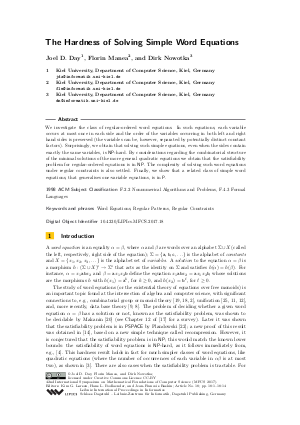Document# The Hardness of Solving Simple Word Equations

### Authors Joel D. Day, Florin Manea, Dirk Nowotka## File

LIPIcs.MFCS.2017.18.pdf
• Filesize: 0.62 MB
• 14 pages

## Cite As

Joel D. Day, Florin Manea, and Dirk Nowotka. The Hardness of Solving Simple Word Equations. In 42nd International Symposium on Mathematical Foundations of Computer Science (MFCS 2017). Leibniz International Proceedings in Informatics (LIPIcs), Volume 83, pp. 18:1-18:14, Schloss Dagstuhl - Leibniz-Zentrum für Informatik (2017)
https://doi.org/10.4230/LIPIcs.MFCS.2017.18

## Abstract

We investigate the class of regular-ordered word equations. In such equations, each variable occurs at most once in each side and the order of the variables occurring in both left and right hand sides is preserved (the variables can be, however, separated by potentially distinct constant factors). Surprisingly, we obtain that solving such simple equations, even when the sides contain exactly the same variables, is NP-hard. By considerations regarding the combinatorial structure of the minimal solutions of the more general quadratic equations we obtain that the satisfiability problem for regular-ordered equations is in NP. The complexity of solving such word equations under regular constraints is also settled. Finally, we show that a related class of simple word equations, that generalises one-variable equations, is in P.
##### Keywords
• Word Equations
• Regular Patterns
• Regular Constraints

## Metrics

• Access Statistics
• Total Accesses (updated on a weekly basis)
0

## References

1. R. Da̧browski and W. Plandowski. Solving two-variable word equations. In Proc. 31th International Colloquium on Automata, Languages and Programming, ICALP 2004, volume 3142 of Lecture Notes in Computer Science, pages 408-419, 2004.2. V. Diekert, A. Jez, and M. Kufleitner. Solutions of word equations over partially commutative structures. In Proc. 43rd International Colloquium on Automata, Languages, and Programming, ICALP 2016, volume 55 of Leibniz International Proceedings in Informatics (LIPIcs), pages 127:1-127:14, 2016.3. V. Diekert and J. M. Robson. On quadratic word equations. In Proc. 16th Annual Symposium on Theoretical Aspects of Computer Science, STACS 1999, volume 1563 of Lecture Notes in Computer Science, pages 217-226, 1999.4. A. Ehrenfeucht and G. Rozenberg. Finding a homomorphism between two words is NP-complete. Information Processing Letters, 9:86-88, 1979.5. H. Fernau, F. Manea, R. Mercaş, and M.L. Schmid. Pattern matching with variables: Fast algorithms and new hardness results. In Proc. 32nd Symposium on Theoretical Aspects of Computer Science, STACS 2015, volume 30 of Leibniz International Proceedings in Informatics (LIPIcs), pages 302-315, 2015.6. H. Fernau and M. L. Schmid. Pattern matching with variables: A multivariate complexity analysis. Information and Computation, 242:287-305, 2015.7. H. Fernau, M. L. Schmid, and Y. Villanger. On the parameterised complexity of string morphism problems. Theory of Computing Systems, 2015. http://dx.doi.org/10.1007/s00224-015-9635-3.8. D. D. Freydenberger. A logic for document spanners. In Proc. 20th International Conference on Database Theory, ICDT 2017, Leibniz International Proceedings in Informatics (LIPIcs), 2017. To appear.9. D. D. Freydenberger and M. Holldack. Document spanners: From expressive power to decision problems. In Proc. 19th International Conference on Database Theory, ICDT 2016, volume 48 of Leibniz International Proceedings in Informatics (LIPIcs), pages 17:1-17:17, 2016.10. M. R. Garey and D. S. Johnson. Computers And Intractability. W. H. Freeman and Company, 1979.11. J. Jaffar. Minimal and complete word unification. Journal of the ACM, 37(1):47-85, 1990.12. A. Jez. Context unification is in PSPACE. In Proc. 41st International Colloquium on Automata, Languages, and Programming, ICALP 2014, volume 8573 of Lecture Notes in Computer Science, pages 244-255. Springer, 2014.13. A. Jeż. One-variable word equations in linear time. Algorithmica, 74:1-48, 2016.14. A. Jeż. Recompression: A simple and powerful technique for word equations. Journal of the ACM, 63, 2016.15. J. Karhumäki, F. Mignosi, and W. Plandowski. The expressibility of languages and relations by word equations. Journal of the ACM, 47:483-505, 2000.16. A. Koscielski and L. Pacholski. Complexity of Makanin’s algorithm. Journal of the ACM, 43(4):670-684, 1996.17. M. Lothaire. Algebraic Combinatorics on Words. Cambridge University Press, Cambridge, New York, 2002.18. R. C. Lyndon. Equations in free groups. Transactions of the American Mathematical Society, 96:445-457, 1960.19. R. C. Lyndon and P. E. Schupp. Combinatorial Group Theory. Springer, 1977.20. G. S. Makanin. The problem of solvability of equations in a free semigroup. Matematicheskii Sbornik, 103:147-236, 1977.21. F. Manea, D. Nowotka, and M. L. Schmid. On the solvability problem for restricted classes of word equations. In Proc. 20th International Conference on Developments in Language Theory, DLT 2016, volume 9840 of Lecture Notes in Computer Science, pages 306-318. Springer, 2016.22. W. Plandowski. An efficient algorithm for solving word equations. In Proceedings of the 38th Annual ACM Symposium on Theory of Computing, STOC 2006, pages 467-476, 2006.23. W. Plandowski and W. Rytter. Application of Lempel-Ziv encodings to the solution of words equations. In Proc. 25th International Colloquium on Automata, Languages and Programming, ICALP'98, volume 1443 of Lecture Notes in Computer Science, pages 731-742. Springer, 1998.24. D. Reidenbach and M. L. Schmid. Patterns with bounded treewidth. Information and Computation, 239:87-99, 2014.25. K. U. Schulz. Word unification and transformation of generalized equations. Journal of Automated Reasoning, 11:149-184, 1995.X

Feedback for Dagstuhl Publishing